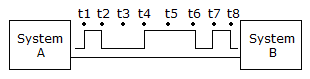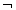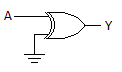# Online Digital Electronics Test - Digital Electronics Test 2Loading Test...

Instruction:

• This is a FREE online test. DO NOT pay money to anyone to attend this test.
• Total number of questions : 20.
• Time alloted : 30 minutes.
• Each question carry 1 mark, no negative marks.
• DO NOT refresh the page.
• All the best :-).

1.

How many binary bits are necessary to represent 748 different numbers?

A.
 9B.
 7C.
 10D.
 82.

SMT stands for small-to-medium technology.

A.
 TrueB.
 False3.

The systems shown in the given figure transfer data ________.A.
 seriallyB.
 sequentiallyC.
 in parallelD.
 both serially and sequentially4.

A.
 8F16B.
 CE16C.
 DF16D.
 CF165.

Zeros may be added to the left of the MSB to produce even groups of 4 bits when converting from binary to hexadecimal.

A.
 TrueB.
 False6.

In binary, the decimal number 93 converts to ________ digits.

A.
 sevenB.
 eightC.
 11D.
 five7.

Which of the following gates has the exact inverse output of the OR gate for all possible input combinations?

A.
 NORB.
 NOTC.
 NANDD.
 AND8.

How many 2-input NOR gates does it take to produce a 2-input NAND gate?

A.
 1B.
 2C.
 3D.
 49.

A digital circuit that converts coded information into a familiar or non-coded form is known as an encoder.

A.
 TrueB.
 False10.

In VHDL, each instance of a component is given a name followed by a semicolon and the name of the library primitive.

A.
 TrueB.
 False11.

The postponed symbol () on the output of a flip-flop identifies it as being ________.

A.
 a D flip-flopB.
 a J-K flip-flopC.
 pulse triggeredD.
 trailing edge-triggered12.

Which of the following procedures could be used to check the parallel loading feature of a counter?

A.
 Preset the LOAD inputs, set the CLR to its active level, and check to see that the Q outputs match the values preset into the LOAD inputs.B.
 Apply LOWs to the parallel DATA inputs, pulse the CLK input, and check for LOWs on all the Q outputs.C.
 Apply HIGHs to all the DATA inputs, pulse the CLK and CLR inputs, and check to be sure that the Q outputs are all LOW.D.
 Apply HIGHs to all the Q terminals, pulse the CLK, and check to see if the DATA terminals now match the Q outputs.13.

To design a divide-by-200 counter using synchronous counters, two 4-bit counters could be cascaded together to form an 8-bit counter.

A.
 TrueB.
 False14.

The refresh period for capacitors used in DRAMs is ________.

A.
 2 msB.
 2sC.
 64 msD.
 64s15.

The output of this circuit is always ________.A.
 1B.
 0C.
 AD.
 A16.

An ALU is a multipurpose device capable of providing several different logic operations.

A.
 TrueB.
 False17.

One of the first steps in any HDL project is to define its scope by naming each input and output.

A.
 TrueB.
 False18.

In the stepper motor, the half-step sequence is used when ________.

A.
 less torque is neededB.
 larger steps are desiredC.
 smaller steps are desirableD.
 more torque is needed19.

What is the difference between the 74HC00 series and the 74HCT00 series of CMOS logic?

A.
 The HCT series is faster.B.
 The HCT series is slower.C.
 The HCT series is input and output voltage compatible with TTL.D.
 The HCT series is not input and output voltage compatible with TTL.20.

Which of the following buses is primarily used to carry signals that direct other ICs to find out what type of operation is being performed?

A.
 data busB.
 control busC.
 address busD.
 address decoder bus